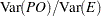Gauge Repeatability and Reproducibility Analysis

In a typical gauge R&R experiment, each operator (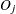) makes multiple observations on each of several similar parts (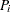) from a monitored process. The statistical model used to describe the response variable is the balanced two-factor crossed random model with interaction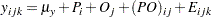where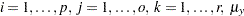is an unknown constant, andare jointly independent normal random variables with means of zero and variances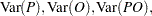and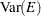, respectively. The corresponding SAS statements are as follows:

```proc varcomp method=grr;
class P O;
model y = P|O;
run;
```

The first random effect in the MODEL statement is assumed to be the "Part" effect and the second is "Operator."

The ANOVA table for the preceding model is shown in Table 97.1.

Table 97.1 GRR Analysis of Variance

Source

DF

Mean Square

Expected Mean Square

Parts(P)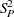Operators(O)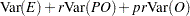PO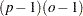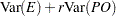Error(E)The gauge R&R parameters of interest are given in Table 97.2 in terms ofand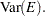Table 97.2 Gauge R&R Parameters

Parameter

Formula

Mean of population of measurements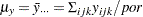Variance of the monitored process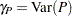Variance of the measurement system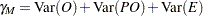Total variance of the response variable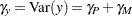Ratio of process variance to measurement varianceProportion of total variance due to the process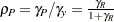Proportion of total variance due to the measurementSignal-to-noise ratio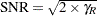Discrimination ratio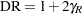For a one-way model,and for a two-way model with no interaction,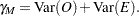If you use the SPECLIMITS option to give specification limits, the two parameters in Table 97.3 will also be estimated and displayed.

Table 97.3 Gauge R&R Parameters Related to Specification Limits

Parameter

Formula

Precision-to-tolerance ratioProcess capability ratio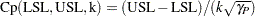Here, USL and LSL are the specification limits, and the valuecorresponds to the number of standard deviations between the "natural" tolerance limits of a normal process.

If you use the RATIO option, the ratios in Table 97.4 will also be estimated and displayed.

Table 97.4 Gauge R&R Ratios

Ratio

Formula

Ratio of process variance to total varianceRatio of operator variance to total variance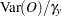Ratio of process by operator variance to total varianceRatio of process variance to residual varianceRatio of operator variance to residual varianceRatio of process by operator variance to residual variance